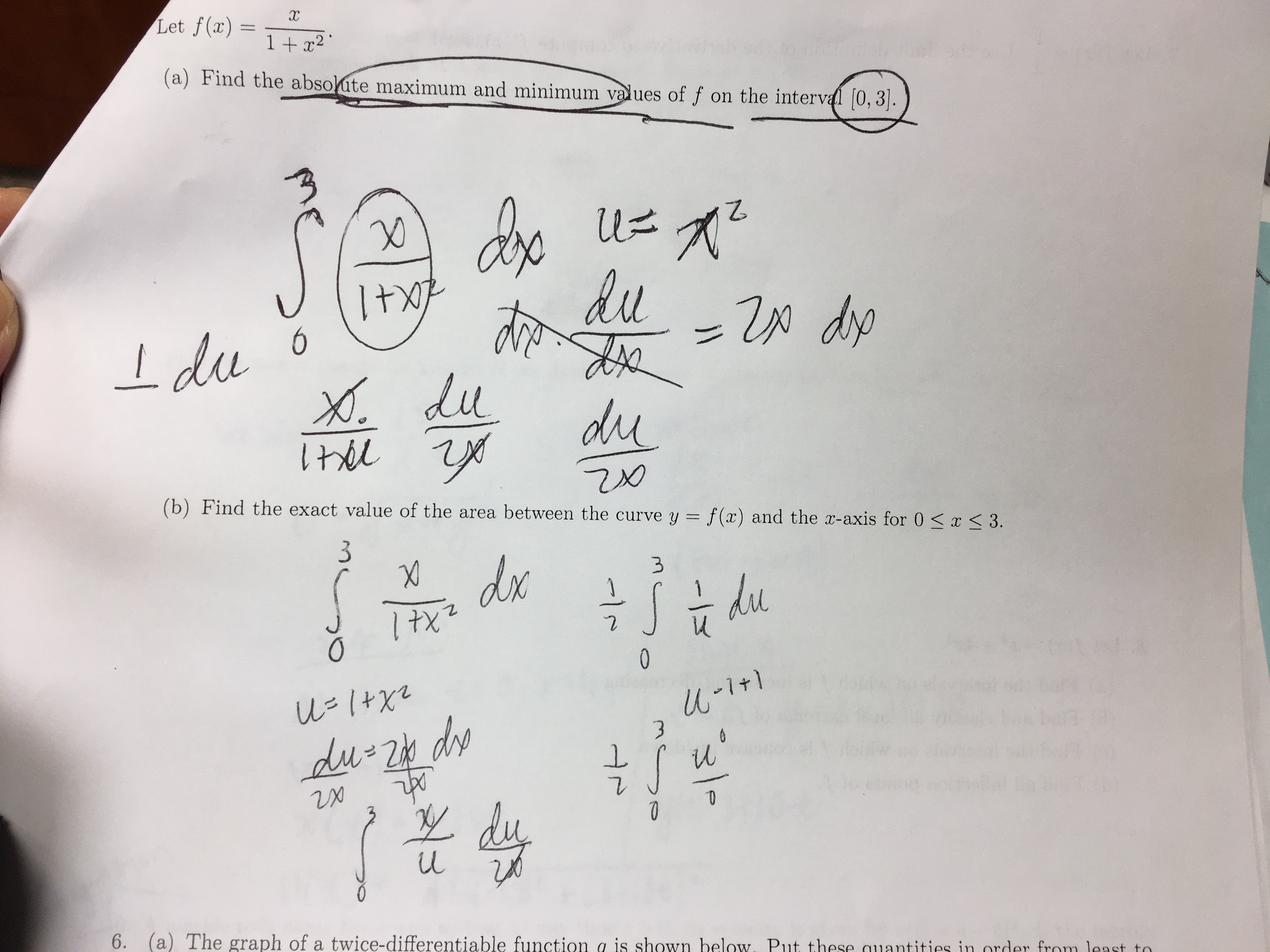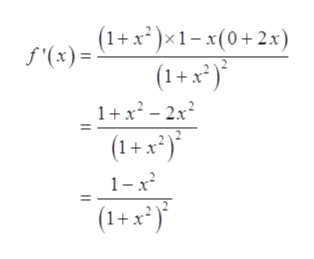# Let f(x)1 + x2 '(a) Find the absolute maximum and minimum values of f on the interval [0, 3].+1duIdu oX.dpdudu(b) Find the exact value of the area between the curve y = f(x) and the x-axis for 0

Question
1 viewshelp_outlineImage TranscriptioncloseLet f(x) 1 + x2 ' (a) Find the absolute maximum and minimum values of f on the interval [0, 3]. +1 du Idu o X. dp du du (b) Find the exact value of the area between the curve y = f(x) and the x-axis for 0
check_circle

Step 1

Given,

Step 2

Part (a):

Find the absolute maximum and minimum values of the function f(x) on the interval [0, 3].

On differentiating with respect to x, it giveshelp_outlineImage Transcriptionclose(1+ x² )×1- x(0+2x) (1+ x*)* f '(x) = 1+x² - 2x? (1+ x*)* 1-x2 (1+x²)* fullscreen
Step 3

Now put f ‘ (...

### Want to see the full answer?

See Solution

#### Want to see this answer and more?

Solutions are written by subject experts who are available 24/7. Questions are typically answered within 1 hour.*

See Solution
*Response times may vary by subject and question.
Tagged in

### Integration